Search the site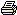Print

 Electrical Laws.
Ohms Law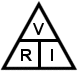V = Volts
R = Resistance (ohms,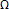)
I = Current ( Amps)Formular Example V = R * I 5* 2A = 10Vs I = V / R 10V / 5= 2A R = V / I 10V / 2A = 5Watts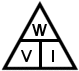W = Watts
V = Volts
I = Current ( Amps)

 Formular Example W = V * I 10V * 2A = 20W I = W / V 20W / 10V = 2A V = W / I 20W / 2A = 10V W = R * I2 5* 22 = 20W W = V2 / R 10V2 / 5= 20W R = W / I2 20W / 2A2 = 5R = V2 / W 10V2 / 20W = 5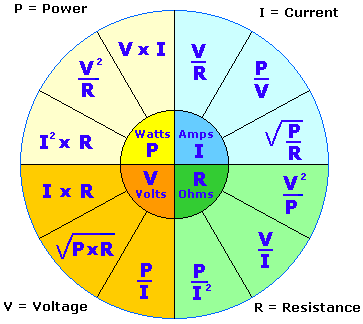AC Theory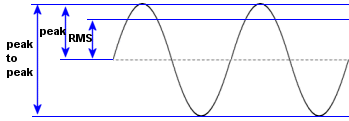RMS 0.707 Peak voltage

Impedance

AC current in a capacitive or inductive component. Impedance is the resistance ( measured in ohms ) of a reactive component ( capacitor, inductor ) at a certain AC frequency.

 Capacitor Inductor Xc = 1 / ( 2 * Pi * f * C ) Xl = 2 * Pi * f * L where Pi = 3.14 where Pi = 3/14 f = frequency in Hz f = frequency in Hz C = capacitance in Farads L = Inductance in Henry eg A 100uF cap at 50Hz eg A 20mH inductor at 50Hz Xc = 1/(2*3.15*50Hx*0.0001F) Xl=2*3.14*50Hx*0.02 Xc = 31.8 ohms Xl = 6.28 ohms